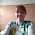## Wednesday, August 31, 2016

### 47 55 68 106 123 | Brazil's first female President could be impeached today, August 31, 2016

Let us take a look at Brazil's first female President, who is facing being impeached.

Right away, her age of 68 stands out.

From today's trip until her 69th birthday is a 106-day span.  Notice below she also has name gematria of '69'.

Notice her place of birth in '47 connects to '106' as well.

Her name connects to Clinton, and so does her year of birth, '47, just like Hillary.  As we know, the NWO likes their agents to be "born to be".

Dilma = 4+9+3+4+1 = 21
Vana = 4+1+5+1 = 11
Rousseff = 9+6+3+1+1+5+6+6 = 37/55
Dilma Vana Rousseff = 69/87 (Clinton = 87)

Dilma = 4+9+12+13+1 = 39 (8/31 = 8+31 = 39)
Vana = 22+1+14+1 = 38
Rousseff = 18+15+21+19+19+5+6+6 = 109
Dilma Vana Rousseff = 186

12/14/1947 = 12+14+19+47 = 92
12/14/1947 = 12+14+(1+9+4+7) = 47 (President = 47)
12/14/1947 = 1+2+1+4+1+9+4+7 = 29
12/14/97 = 12+14+97 = 123 (Conspiracy)

Today's date connects to the last name 'Rousseff' as well.

Rousseff = 9+6+3+1+1+5+6+6 = 37/55

If you go the CNN story linked, you'll see there video is 1:06.

http://www.cnn.com/2016/08/31/americas/brazil-rousseff-impeachment-vote/index.html

Two final points.

One, this news comes right after Rio hosted the Summer Olympics, beginning August 5.

Also, Richard Nixon was once impeached August 9, 1974.  This woman is impeached on the last day of August.

1.Zach you forgot to update your thoughts on World Series on Aug 29, 57 days before World Series

1.Thank you Mike, I actually appreciate this post.

2.2.